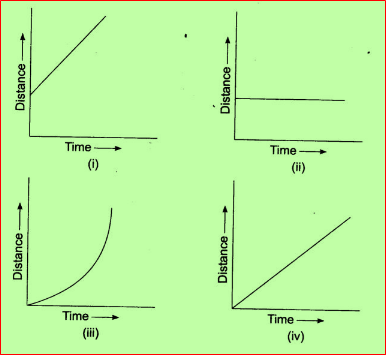# NCERT Class VII: Science-Exercise Solutions

### Chapter 13-Motion and Time-Exercise Solutions

NCERT Book Page Number- 156
Q.1. Classify the following as motion along a straight line, circular or oscillatory motion:
(i) Motion of your hands while running.
(ii) Motion of a horse pulling a cart on a straight road.
(iii) Motion of a child in a merry-go-round.
(iv) Motion of a child on a see-saw.
(v) Motion of the hammer of an electric bell.
(vi) Motion of a train on a straight bridge.
(i) Motion of your hands while running – Oscillatory motion.
Explanation: –
During running the hands move to and fro and as this motion gets repeated after certain time interval.
Therefore it is oscillatory motion.
(ii) Motion of a horse pulling a cart on a straight road – Straight line motion.
Explanation: –
As the horse cart is moving on a straight road. Therefore motion is along the straight line.
(iii) Motion of a child in a merry-go-round – Circular motion.
Explanation: –
Motion of the merry-go-round is circular. Therefore kids sitting inside it experiences the circular motion.
(iv) Motion of a child on a see-saw – Oscillatory motion.
Explanation: –
As the see-saw goes up and down continuously .Therefore it is oscillatory motion.
(v) Motion of the hammer of an electric bell – Oscillatory motion.
Explanation: –
When the hammer vibrates the bell it starts vibrating. It is an example of oscillatory motion.
(vi) Motion of a train on a Straight Bridge – Straight line motion.
Explanation: –
The train is moving on the straight bridge. It exhibits motion of a straight line.

Q.2. Which of the following are not correct?
(i) The basic unit of time is second.
(ii) Every object moves with a constant speed.
(iii) Distances between two cities are measured in kilometres.
(iv) The time period of a given pendulum is not constant.
(v) The speed of a train is expressed in m/h.
(i) The basic unit of time is second – Correct
Explanation: –
SI unit of time is second.
(ii) Every object moves with a constant speed – Not correct
Explanation: –
Speed of object is constant or variable.
(iii) Distances between two cities are measured in kilometres – Correct
Explanation: –
The distance between two cities is very large. And as a kilometre is a bigger unit therefore it is used
to measure the distance between two cities.
(iv) The time period of a given pendulum is not constant – Not correct
Explanation: –
The time period depends upon the length of the thread. Therefore it will be constant for a particular pendulum.
(v) The speed of a train is expressed in m/h – Not correct
Explanation: –
The speed of train is measured either in km/h or m/s.

Q.3. A simple pendulum takes 32s to complete 20 oscillations. What is the time period of the pendulum?
Answer: The time taken to complete one oscillation is known as time period of the pendulum.
$Time Period = \frac{Total time taken}{Number of Oscillations}$
Given: – 20 oscillations taking 32s to complete.
Therefore 1 oscillation will take = $\frac{32}{20}$ sec = 1.6 second
Therefore 1.6s is the time period of the pendulum.

Q.4. The distance between two stations is 240 km. A train takes 4 hours to cover this distance.
Calculate the speed of the train.
Answer: $\Speed=frac{Distance travelled}{ Time}$
$= \frac{240 km}{4h}$=60 Kmh^{-1}$Therefore the speed of the train is$=60 Kmh^{-1}$. Q.5. The odometer of a car reads 57321.0 km when the clock shows the time 08:30 AM. What is the distance moved by the car, if at 08:50 AM, the odometer reading has changed to 57336.0 km? Calculate the speed of the car in km/min during this time. Express the speed in km/h also. Answer: Initial reading of the odometer of the car =57321.0 km Final reading of the odometer of the car = 57336.0 km The car starts at 8:30 AM and stops at 8:50 AM. Distance covered by car = (57336 – 57321) km = 15 km Time taken between 08:30 AM to 08:50 AM = 20 minutes =$\frac{20}{60}$hour =$\frac{1}{3} hour$So Speed in km/min$ Speed = \frac{Distance travelled}{Time} = \frac{15km}{20min} =0.75km min^{-1}$Speed in km/h$Speed =\frac{Distance travelled}{Time}= \frac{15km}{(1/3h}= \frac{(15 × 3) km}{1h}$=45km/h Q.6. Salma takes 15 minutes from her house to reach her school on a bicycle. If the bicycle has a speed of 2 m/s, calculate the distance between her house and the school. Answer: Speed = 2m/s Time taken to reach school = 15 minutes = 15 × 60 seconds = 900 seconds$Speed = \frac{Distance travelled}{Time}$Distance = (Speed x Time) =2 × 900 =1800 m Also 1km= 1000m Therefore 1800 ×$\frac{1}{1000}$= 1.8km. The distance between her house and the school is 1.8 km. Q.7. Show the shape of the distance-time graph for the motion in the following cases: (i) A car moving with a constant speed. (ii) A car parked on a side road. Answer: (i) A car moving with a constant speed covers equal distance in equal intervals of time. It will be a uniform motion. Distance-time graph will be as below: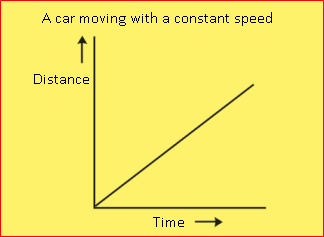(ii) A car parked on a road there is no change in the distance with the time. No motion. Therefore the graph so obtained will be parallel to x-axis. Distance- time graph will be as below:-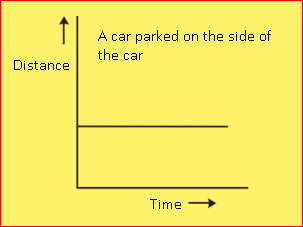Q.8. Which of the following relations is correct? (i) Speed = (Distance × Time) (ii)$ Speed = \frac{Distance}{Time}$(iii)$Speed = \frac{Time}{Distance}$(iv)$ Speed = \frac{1}{ (Distance ×Time}$Answer: Speed of an object is given by the relation:- (ii)$Speed =\frac{Distance}{Time} $NCERT Book Page Number-157 Q.9. The basic unit of speed is: (i) km/min (ii) m/min (iii) km/h (iv) m/s Answer: (iv) m/s. The unit of distance is metre (m) and of time is second(s).$Speed = \frac{Distance}{Time}$Therefore the basic unit of speed is m/s. Q.10. A car moves with a speed of 40 km/h for 15 minutes and then with a speed of 60 km/h for the next 15 minutes. The total distance covered by the car is: • 100 km • 25 km • 15 km • 10km Answer: (ii) 25 km Case I: Speed = 40 km/h Time = 15 min =$\frac{15}{60}$hour Distance (d1) = Speed × Time = 40 ×$\{\frac{15}{60}$= 10 km Case II: Speed = 60 km/h$Time = 15 min = \frac{15}{60}$hour Distance$ (d_{2} = (Speed × Time) = 60 × \frac{15}{60}$= 15 km Total distance (d) =$ (d_{1} + d_{2}\$) = 10 km + 15 km = 25 km
Therefore the total distance covered by the car = 25km.

Q.11. Suppose the two photographs, shown in Fig (1) and Fig (2), had been taken at an interval of 10 seconds.
If a distance of 100 metres is shown by 1 cm in these photographs, calculate the speed of the blue car.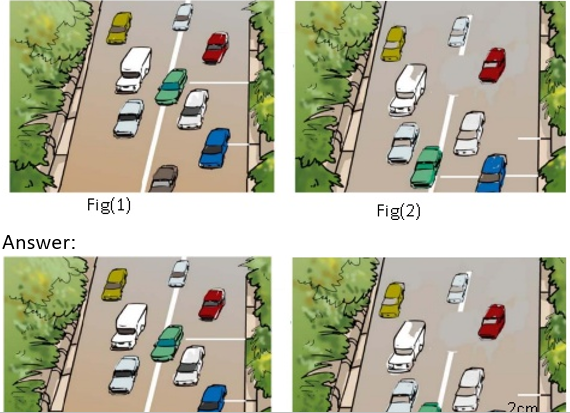With the help of the scale we will first measure the distance.
Suppose the distance measured is 2cm.
So, the distance covered d = 2 x 100= 2m. (Because 1m=100cm).
Time taken = 10seconds.
Speed = (Distance/Time) = (200m/10s) = 20m/s.
Therefore the speed of the blue car = 20m/s.

Q.12. Fig (3) shows the distance-time graph for the motion of two vehicles A and B. Which one of them is moving faster?
Fig (3):- Distance– time graph for the motion of two cars.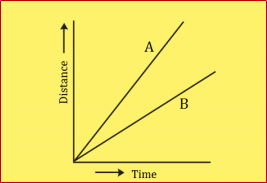Answer: In distance – time graph speed is measured by its slope.
Vehicle A is moving faster as the slope of the graph of A is more than the slope of the graph B.

Q.13. Which of the following distance-time graphs shows a truck moving with speed which is not constant?